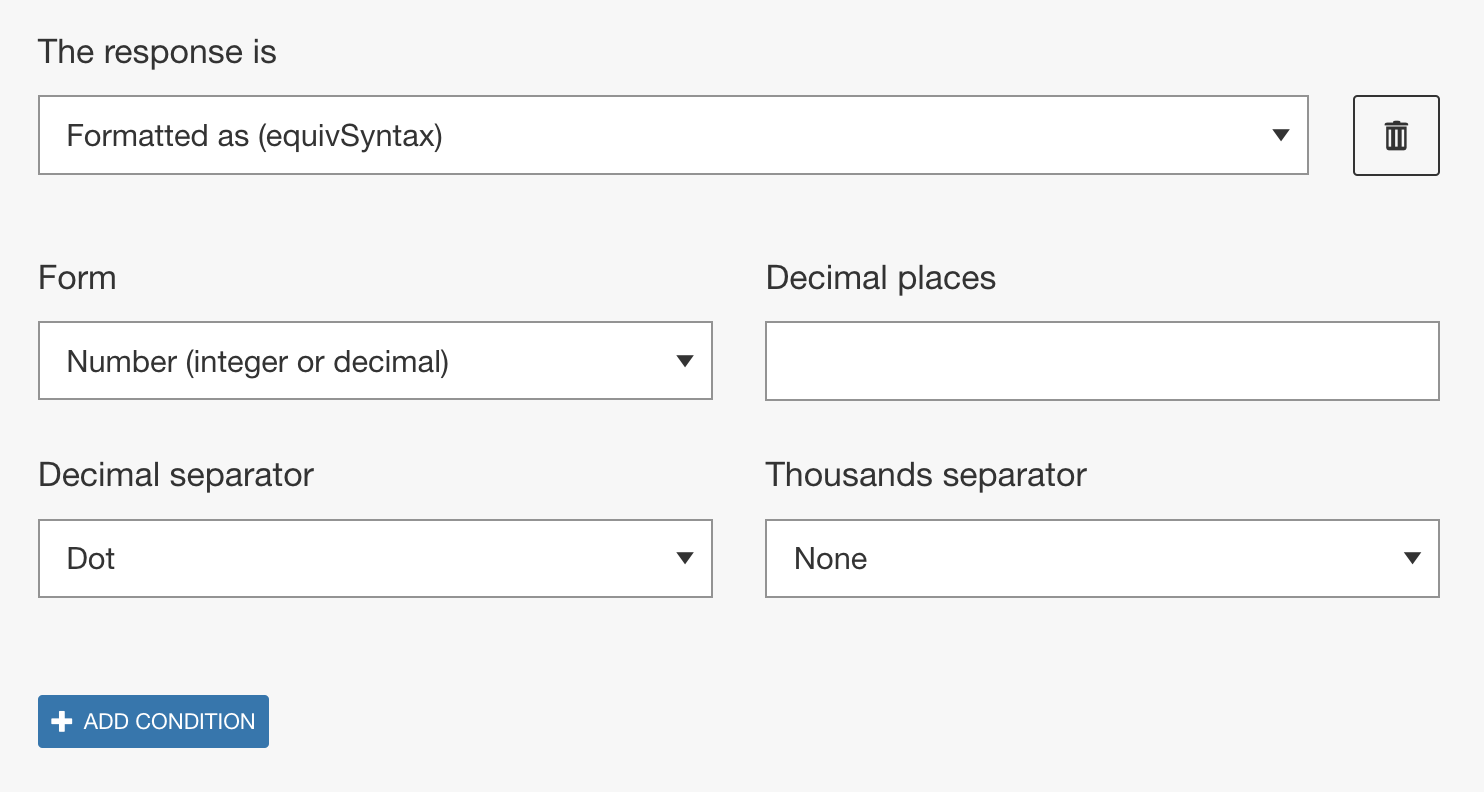# Formatted as (equivSyntax)

## Overview

The Formatted as (equivSyntax) method checks if the learner response is expressed in a specific format. This method is not a comparison method so no correct answer is set by the author. Instead when this method is used alone, any answer that is of the specified form will be marked as correct.

When combined with the Numerically equals to (equivValue) method, this method can be useful for constraining the possible correct answers.Forms
There are eight different forms that you can select from. Some forms have an additional field associated with them that allows you to further define the desired correct answer format. We are using the convention that all non-zero digits, all trailing zeros, and zeros between non-zero digits are significant and that all leading zeros are not significant.

• Number (integer or decimal) - Any integer or floating point.
• Integer - Any integer.
• Decimal - Any decimal value.
• Scientific - Any number in scientific notation. This option requires the coefficient to be greater than or equal to one and less than 10.
• Fraction - Any fraction, either a simple or mixed fraction.
• Mixed fraction - Any mixed fraction. This option requires the numerator of the fractional part to be less than the denominator.
• Simple fraction - Any fraction expressed as an integer divided by an integer.
• Fraction or decimal - Any fraction or decimal.

Decimal and thousands separator
Authors can specify what separators should be recognized by the engine and can be used by the learner. From the Decimal separator drop-down either a dot or a comma can be selected. Depending on the selection of the decimal separator, from the Thousand Separator drop-down dot, comma, and/or space can be selected.

## Examples

 Form Additional field Learner response Result Number 2 0.5 .5 .500 .834 1/2 true true true true true false Number 1 0.5 .5 .500 true true false Integer 5 0.50 1÷2 50% true false false false Integer 3 105 100 1000 true true false Decimal 1.0 1.23 1. 1 true true true false Decimal 3 1.000 1.234 1.2 true true false Scientific 2 \times 10^3 1.2 \times 10^{-2} 10 \times 10^2 true true false Scientific 2 2.34 \times 10^3 1.2 \times 10^{-2} false true